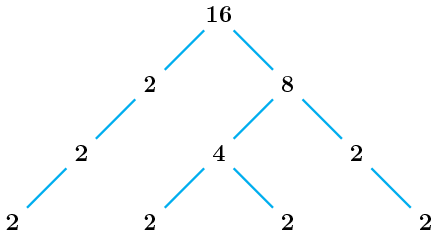# RATL 2 | Lesson 1 | Solutions (Prime Factorization)

For extra help, here are some strategies for prime factoring whole numbers.

1. The first way is to use a table as shown in this slide show.
2. Another way to prime factor a composite number is to use a factor ladder:Prime factorization for 48:  $$48=2^4\cdot{3}$$

1. A third way to prime factor a number is to use a factor tree.1. $$\dfrac{24}{36}=\dfrac{2\cdot{2}\cdot{2}\cdot{3}}{2\cdot{2}\cdot{3}\cdot{3}}=\dfrac{2}{3}$$
2. $$\dfrac{48}{54}=\dfrac{2\cdot{2}\cdot{2}\cdot{2}\cdot{3}}{2\cdot{3}\cdot{3}\cdot{3}}=\dfrac{2\cdot{2}\cdot{2}}{3\cdot{3}}=\dfrac{8}{9}$$
3. $$\dfrac{36}{54}=\dfrac{2\cdot{2}\cdot{3}\cdot{3}}{2\cdot{3}\cdot{3}\cdot{3}}=\dfrac{2}{3}$$
4. $$\dfrac{24}{48}=\dfrac{2\cdot{2}\cdot{2}\cdot{3}}{2\cdot{2}\cdot{2}\cdot{2}\cdot{3}}=\dfrac{1}{2}$$